# Introduction

The collection interfaces are divided into two groups: the most basic interface java.util.Collection, and other collection interfaces based on java.util.Map. In this section, we will cover four main interfaces in the Java Collections Framework: List, Set, Map, and Queue.

# List

You usually use a list when you want an ordered collection that can contain duplicate entries. All list methods are ordered and allow duplicates.

## ArrayList

When to use: Use when you are not sure which collection to use and/or when you are reading more often than writing to the ArrayList
Pros: Lookup is constant time, O(1)
Cons: Adding and removing is slower. The add operation runs in amortized constant time, that is, adding n elements requires O(n) time

`List<String> list = new ArrayList<>(); List<Integer> list = new ArrayList<>(); ...`

When to use: LinkedList is usually used as a Queue
Pros: You can access, add, and remove from the beginning and end of the list in constant time, O(1)
Cons: Dealing with an arbitrary index takes linear time, O(n)

`LinkedList<String> linkedList = new LinkedList<String>();`

## Stack

When to use: A Stack is a data structure that you add and remove elements from the top of the stack. It is usually used as an ArrayDeque
Pros: The Stack class represents a last-in-first-out (LIFO) stack of objects
Cons: Elements are not sorted

`Deque<String> stack = new ArrayDeque<>();stack.push("1"); stack.push("2"); stack.pop(); // 2`

## List Methods

The List methods that you need to know are listed below:

`void add(E element)void add(int index, E element)E get(int index)int indexOf(Object o)int lastIndexOf(Object o)void remove(int index)E set(int index, E e)`

This example shows the usage of list methods:

`List<String> list = new ArrayList<>(); list.add("suleyman"); // suleyman list.add(1,"yildirim"); // [suleyman, yildirim] list.get(1); //yildirim //list.set(2, "asd"); // java.lang.IndexOutOfBoundsException list.set(1, "DDD"); //[suleyman, DDD] list.indexOf("DDD"); // 1 list.lastIndexOf("suleyman"); // 1 list.remove(0); // [DDD] list.remove("DDD"); // []`

# Set

A collection that contains no duplicate elements. You use a set when you don’t want to allow duplicate entries.

## HashSet

A HashSet implements the Set interface, backed by a hash table (actually a HashMap instance). It stores its elements in a hash table.

Pros: This class offers constant time performance for the basic operations (add, remove, contains and size)
Cons: It makes no guarantees as to the iteration order of the set; in particular, it does not guarantee that the order will remain constant over time.

`Set<String> hashSet = new HashSet<>();hashSet.add("suleyman");hashSet.add("canan");hashSet.add("fatma");hashSet.add("omur");hashSet.add("fatma");System.out.println("HashSet values:");for (String value : hashSet) {    System.out.print(value + " ");}// HashSet values: Canan Suleyman Fatma Omur`

## TreeSet

A TreeSet stores its elements in a sorted tree structure. It implements the NavigableSet interface.

Pros: The set is always in sorted order
Cons: Adding and checking if an element is present are both O(log n)

`Set<String> treeSet = new TreeSet<>();treeSet.add("suleyman");treeSet.add("canan");treeSet.add("fatma");treeSet.add("omur");treeSet.add("fatma");System.out.println("TreeSet values:");for (String value : treeSet) {    System.out.print(value + " ");}// TreeSet values: Canan Fatma Omur Suleyman`

# Queue

A collection designed for holding elements prior to processing. Queues typically, but do not necessarily, order elements in a FIFO (first-in-first-out) manner.

LinkedList implements both the List and Queue interfaces. It is a double-ended queue.

When to use: LinkedList is usually used as a Queue
Pros: You can access, add, and remove from the beginning and end of the list in constant time, O(1)
Cons: Dealing with an arbitrary index takes linear time, O(n)

`LinkedList<String> linkedList = new LinkedList<String>();`

## ArrayDeque

A “pure” double-ended queue and a resizable-array implementation of the interface. Array deques have no capacity restrictions; they grow as necessary to support usage.

When to use: ArrayDeque is usually used as LIFO (Last-In-First-Out) stacks
Pros: Faster than when used as a stack and faster than `LinkedList` when used as a queue
Cons: Remove operation runs in linear time

`Deque<String> stack = new ArrayDeque<>();stack.push("1");stack.push("2");`

## Queue Methods

You need to know the methods below in addition to the ones inherited from the Collection interface. These methods are shown below:

`boolean add(E e)  E element()  boolean offer(E e)  E remove()  void push(E e)  E poll()  E peek()  E pop()`

This example shows the usage of Queue methods:

`Queue<Integer> queue = new ArrayDeque<>();queue.add(1); // queue.add(2); // [1, 2]queue.offer(3); // [1, 2, 3]queue.offer(4); // [1, 2, 3, 4]queue.poll(); // [2, 3, 4]queue.peek(); // [2, 3, 4]queue.poll(); // 3, 4]`

# Map

An object that maps keys to values. A map cannot contain duplicate keys; each key can map to at most one value. You use a map when you want to identify values by a key.

## HashMap

Hash table based implementation of the Map interface. A HashMap stores the keys in a hash table.

Pros: Adding elements and retrieving the element by key both have a constant time
Cons: You lose the order in which you inserted the elements. If you are concerned with this, use LinkedHashMap

`Map<String, Integer> hashMap = new HashMap<>();hashMap.put("11", 10);hashMap.put("gfds", 20);hashMap.put("gnh", 30);hashMap.put("100", 30);hashMap.put("567", 40);hashMap.put("hjk", 50);hashMap.put("aa", 60);hashMap.put("bf", 70);System.out.println(hashMap); // {11=10, gnh=30, aa=60, 100=30, bf=70, 567=40, hjk=50, gfds=20}`

## TreeMap

A Red-Black tree-based `NavigableMap` implementation. A TreeMap stores the keys in a sorted tree structure

Pros: The keys are always in sorted order.
Cons: Adding and checking if a key is present are O(log n)

Note that Integer keys are tricky in TreeMap. That’s why the element (100,30) showed up before (11,10):

`Map<String, Integer> treeMap = new TreeMap<>();treeMap.put("11", 10);treeMap.put("gfds", 20);treeMap.put("gnh", 30);treeMap.put("100", 30);treeMap.put("567", 40);treeMap.put("hjk", 50);treeMap.put("aa", 60);treeMap.put("bf", 70);System.out.println(treeMap); // {100=30, 11=10, 567=40, aa=60, bf=70, gfds=20, gnh=30, hjk=50}`

## Map Methods

You need to know the following methods:

`void clear()boolean isEmpty() int size() V get(Object key) V put(K key, V value)V remove(Object key) containsKey(Object key)containsValue(Object)Set<K> keySet()Collection<V> values()`

In this example, we use some of the Map methods to calculate the frequency of each word in a String list:

`String[] str = {"aaa", "bb", "aaa", "c", "bb", "aaa"};Map<String, Integer> map = new HashMap<>();for (String value : str) {    if (!map.containsKey(value)) {        map.put(value, 1);    } else {        int frequency = map.get(value);        map.put(value, ++frequency);    }}for (String key : map.keySet()) {    System.out.print(key + ", "); //aaa, bb, c,}for (Integer value : map.values()) {    System.out.print(value + ", "); // 3, 2, 1,}`

# Summary

In this section, we covered four main interfaces in the Java Collections Framework: List, Set, Map, and Queue. You can find the source code on GitHub.

Originally published at http://suleymanyildirim.org on January 18, 2020.

## More from Suleyman Yildirim

Software Engineer, Oracle Certified Java Programmer, http://suleymanyildirim.org/

Love podcasts or audiobooks? Learn on the go with our new app.

## Extracting a New Class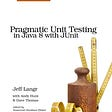## Keep a Finger on the Pulse## Counting Calories Over Follo wing Trends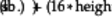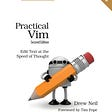## Use Renovate to Manage Dependencies in Gitlab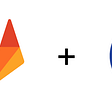## Top 10 GitHub best practices for developers## Suleyman Yildirim

Software Engineer, Oracle Certified Java Programmer, http://suleymanyildirim.org/

## Code structure in Java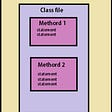## Collection Framework in Java## JVM vs JDK vs JRE